# Solving Equations Inequalities Objectives To solve equations To

• Slides: 19Solving Equations & Inequalities Objectives: To solve equations, To solve problems by writing equations, To solve and graph inequalities, To write and solve compound inequalities Essential Understanding: You can use the properties of equality to solve equations. You can use the properties of inequality to solve inequalities.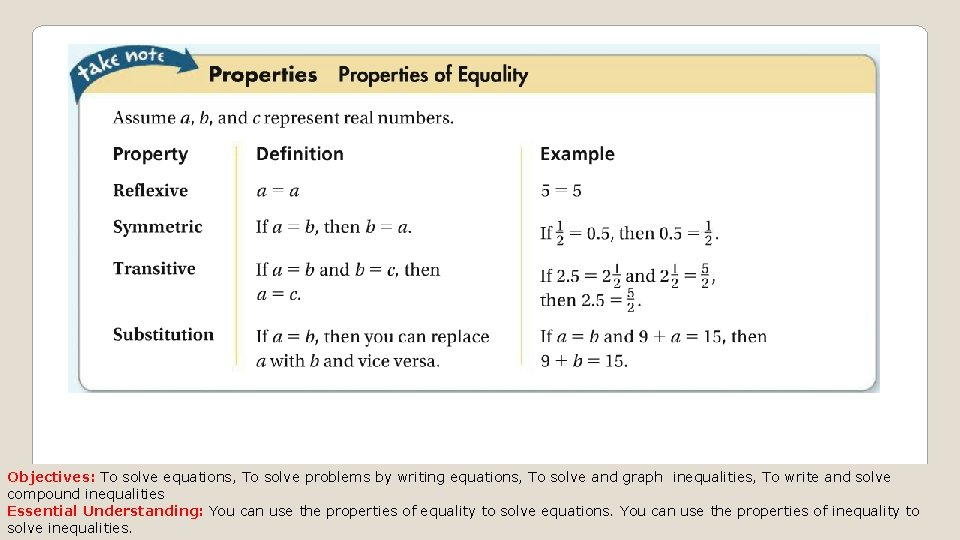Objectives: To solve equations, To solve problems by writing equations, To solve and graph inequalities, To write and solve compound inequalities Essential Understanding: You can use the properties of equality to solve equations. You can use the properties of inequality to solve inequalities.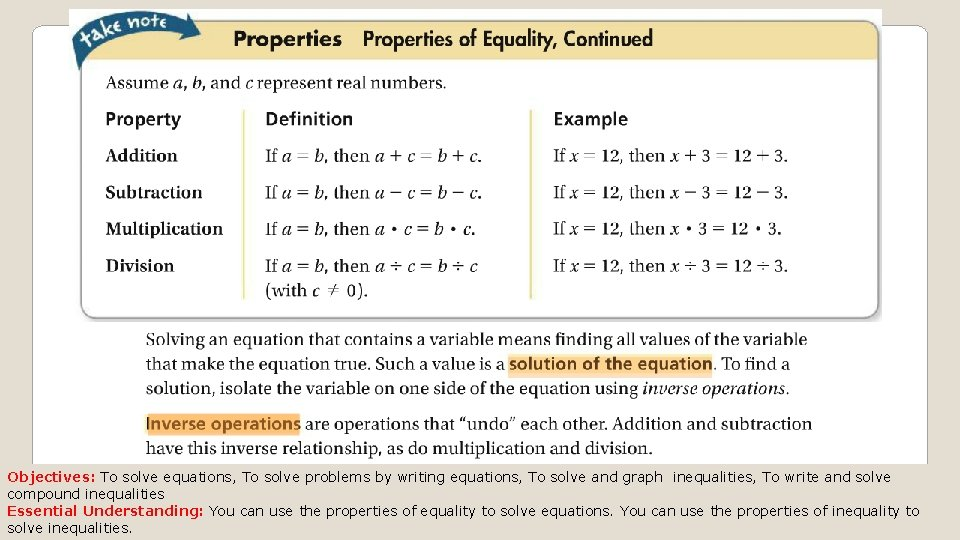Objectives: To solve equations, To solve problems by writing equations, To solve and graph inequalities, To write and solve compound inequalities Essential Understanding: You can use the properties of equality to solve equations. You can use the properties of inequality to solve inequalities.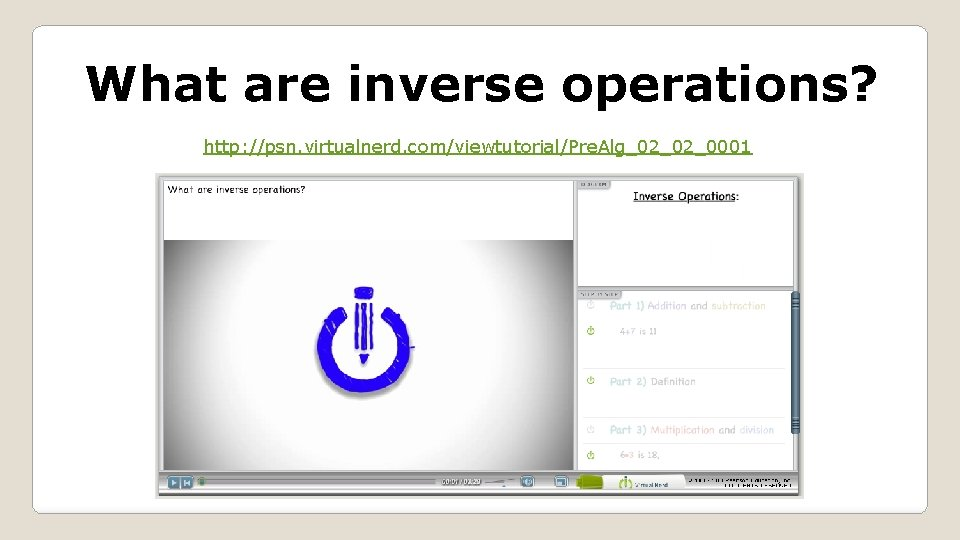What are inverse operations? http: //psn. virtualnerd. com/viewtutorial/Pre. Alg_02_02_0001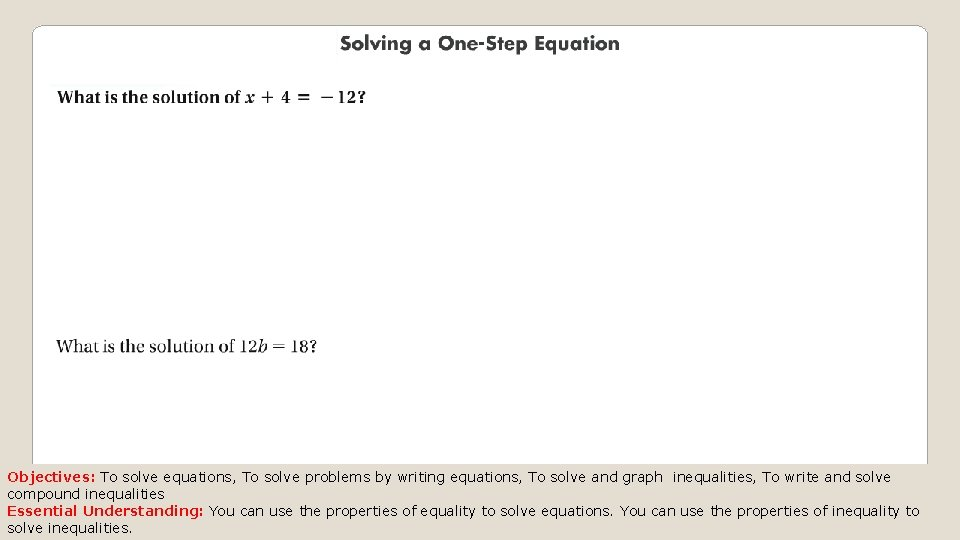Objectives: To solve equations, To solve problems by writing equations, To solve and graph inequalities, To write and solve compound inequalities Essential Understanding: You can use the properties of equality to solve equations. You can use the properties of inequality to solve inequalities.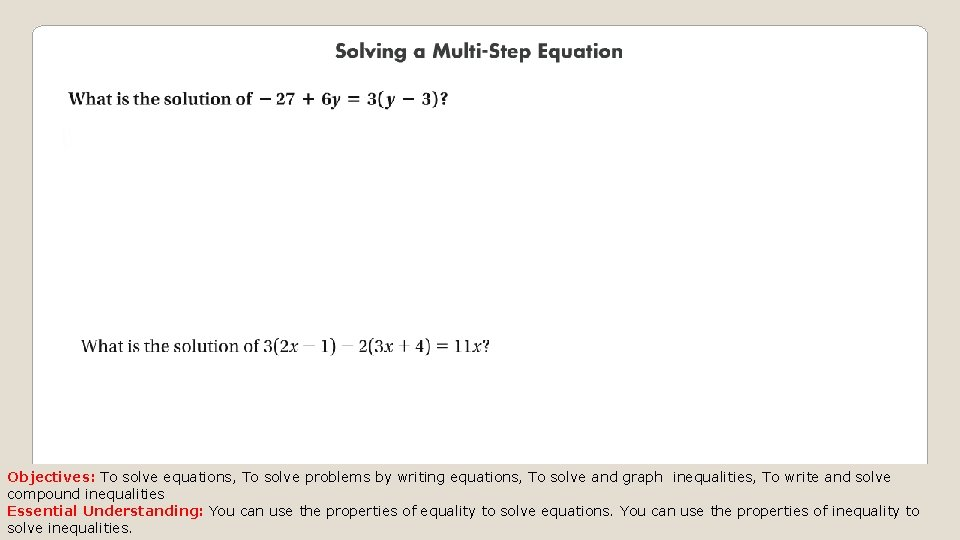Objectives: To solve equations, To solve problems by writing equations, To solve and graph inequalities, To write and solve compound inequalities Essential Understanding: You can use the properties of equality to solve equations. You can use the properties of inequality to solve inequalities.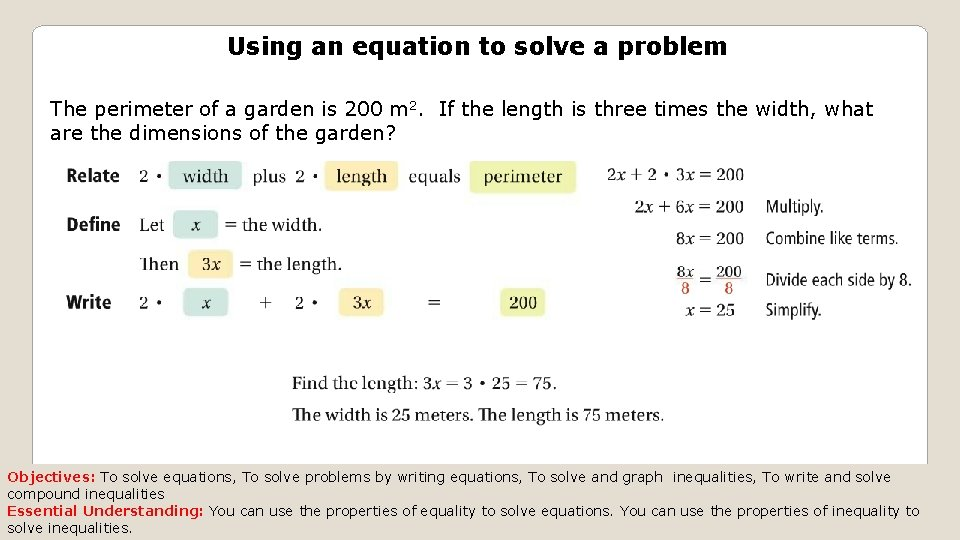Using an equation to solve a problem The perimeter of a garden is 200 m 2. If the length is three times the width, what are the dimensions of the garden? Objectives: To solve equations, To solve problems by writing equations, To solve and graph inequalities, To write and solve compound inequalities Essential Understanding: You can use the properties of equality to solve equations. You can use the properties of inequality to solve inequalities.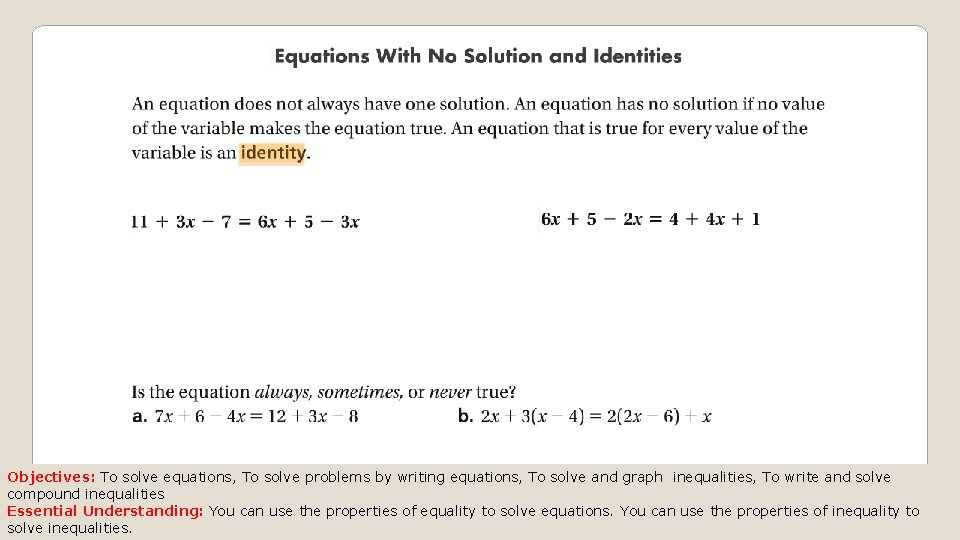Objectives: To solve equations, To solve problems by writing equations, To solve and graph inequalities, To write and solve compound inequalities Essential Understanding: You can use the properties of equality to solve equations. You can use the properties of inequality to solve inequalities.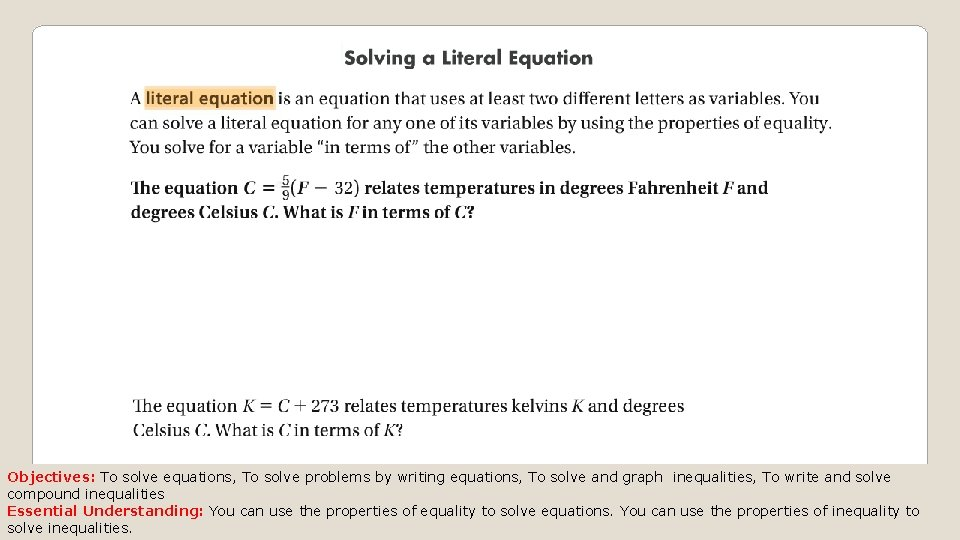Objectives: To solve equations, To solve problems by writing equations, To solve and graph inequalities, To write and solve compound inequalities Essential Understanding: You can use the properties of equality to solve equations. You can use the properties of inequality to solve inequalities.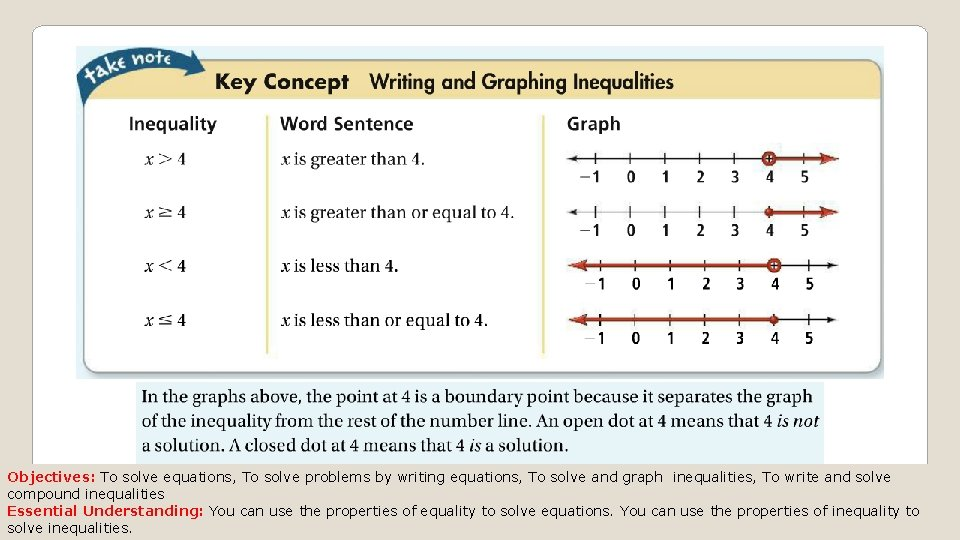Objectives: To solve equations, To solve problems by writing equations, To solve and graph inequalities, To write and solve compound inequalities Essential Understanding: You can use the properties of equality to solve equations. You can use the properties of inequality to solve inequalities.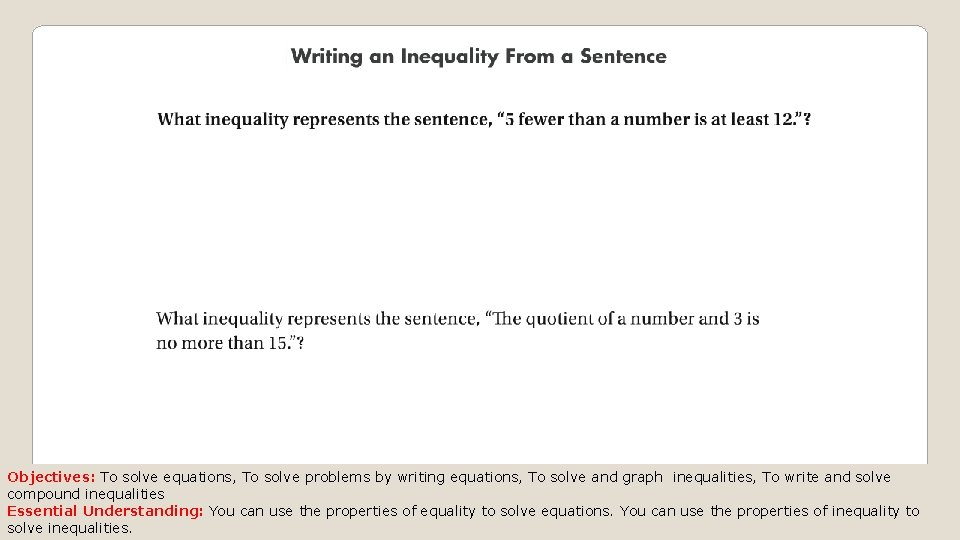Objectives: To solve equations, To solve problems by writing equations, To solve and graph inequalities, To write and solve compound inequalities Essential Understanding: You can use the properties of equality to solve equations. You can use the properties of inequality to solve inequalities.Objectives: To solve equations, To solve problems by writing equations, To solve and graph inequalities, To write and solve compound inequalities Essential Understanding: You can use the properties of equality to solve equations. You can use the properties of inequality to solve inequalities.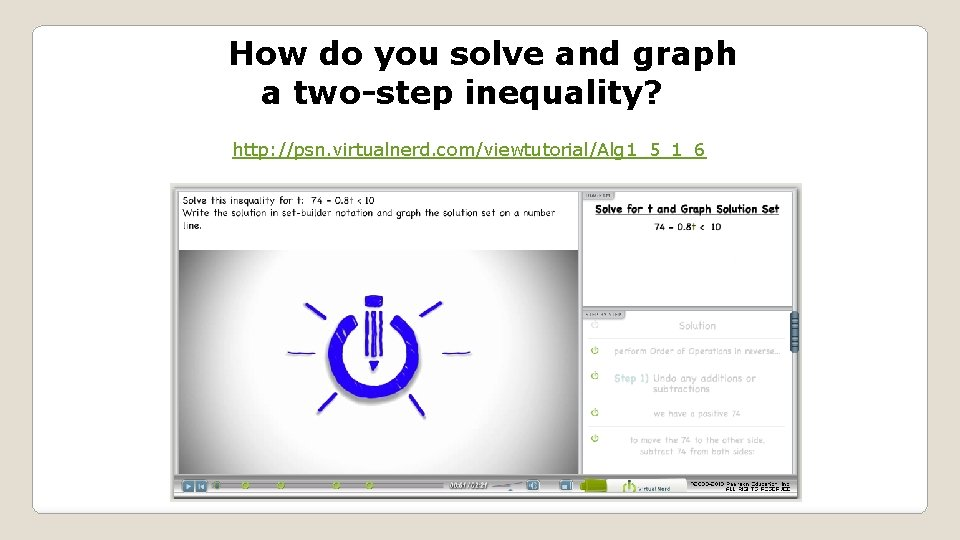How do you solve and graph a two-step inequality? http: //psn. virtualnerd. com/viewtutorial/Alg 1_5_1_6Objectives: To solve equations, To solve problems by writing equations, To solve and graph inequalities, To write and solve compound inequalities Essential Understanding: You can use the properties of equality to solve equations. You can use the properties of inequality to solve inequalities.Objectives: To solve equations, To solve problems by writing equations, To solve and graph inequalities, To write and solve compound inequalities Essential Understanding: You can use the properties of equality to solve equations. You can use the properties of inequality to solve inequalities.Objectives: To solve equations, To solve problems by writing equations, To solve and graph inequalities, To write and solve compound inequalities Essential Understanding: You can use the properties of equality to solve equations. You can use the properties of inequality to solve inequalities.Objectives: To solve equations, To solve problems by writing equations, To solve and graph inequalities, To write and solve compound inequalities Essential Understanding: You can use the properties of equality to solve equations. You can use the properties of inequality to solve inequalities.Objectives: To solve equations, To solve problems by writing equations, To solve and graph inequalities, To write and solve compound inequalities Essential Understanding: You can use the properties of equality to solve equations. You can use the properties of inequality to solve inequalities.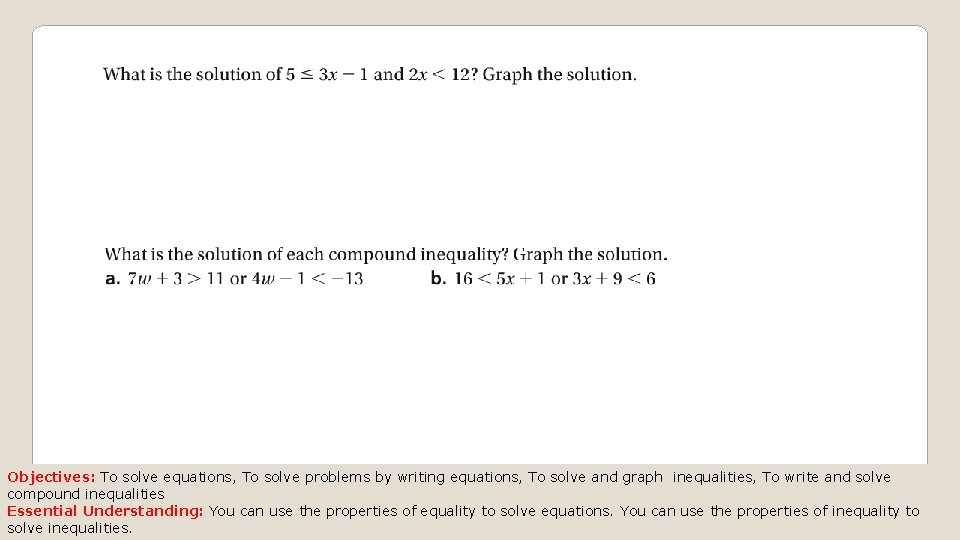Objectives: To solve equations, To solve problems by writing equations, To solve and graph inequalities, To write and solve compound inequalities Essential Understanding: You can use the properties of equality to solve equations. You can use the properties of inequality to solve inequalities.